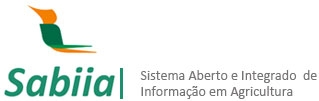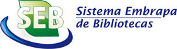Autor
 Palavra-chave
 Tipo do documento
 Ano
 País
 Idioma
 Ordenar por: Relevância Autor Título AnoPrimeira ... 1 ... ÚltimaBatista,Ben Dêivide de Oliveira; Ferreira,Daniel Furtado; Chaves,Lucas Monteiro. ABSTRACT The distribution of externally studentized midrange was created based on the original studentization procedures of Student and was inspired in the distribution of the externally studentized range. The large use of the externally studentized range in multiple comparisons was also a motivation for developing this new distribution. This work aimed to derive analytic equations to distribution of the externally studentized midrange, obtaining the cumulative distribution, probability density and quantile functions and generating random values. This is a new distribution that the authors could not find any report in the literature. A second objective was to build an R package for obtaining numerically the probability density, cumulative distribution and... Tipo: Info:eu-repo/semantics/article Palavras-chave: Distribution function; Density function; Gauss-Legendre quadrature; Newton-Raphson method; R.. Ano: 2017 URL: http://www.scielo.br/scielo.php?script=sci_arttext&pid=S1413-70542017000400378Sheremeta, Roman; Kuzminskyj, Roman. Dimensions of 100 randomly selected coffee beans of the Arabica and Robusta variety were determined by measuring their length (l), width (b) and thickness (h). The results of the measurements were processed by the methods of mathematical statistics. Parameters of distributions of separate sizes as random variables are determined. By the value of the coefficient of variation, the density function of normal distribution (Gaussian distribution) is taken as a model of separate sizes of beans. Models of two-dimensional distributions of beans sizes as independent random variables are presented. The coefficients of correlation between the geometric sizes of beans are calculated. The obtained values of the correlation coefficients indicate that the geometric sizes... Tipo: Info:eu-repo/semantics/article Palavras-chave: Coffee beans; Geometric parameters; Mathematical models; Distribution function. Ano: 2019 URL: http://www.cigrjournal.org/index.php/Ejounral/article/view/5018Cox, Nicholas J.. Graphing univariate distributions is central to both statistical graphics, in general, and Stata’s graphics, in particular. Now that Stata 8 is out, a review of official and user-written commands is timely. The emphasis here is on going beyond what is obviously and readily available, with pointers to minor and major trickery and various user-written commands. For plotting histogram-like displays, kernel-density estimates and plots based on distribution functions or quantile functions, a large variety of choices is now available to the researcher. Tipo: Journal Article Palavras-chave: Graphics; Histogram; Spikeplot; Dotplot; Onewayplot; Kdensity; Distplot; Qplot; Skewplot; Bin width; Rug; Density function; Kernel estimation; Transformations; Logarithmic scale; Root scale; Intensity function; Distribution function; Quantile function; Skewness; Research Methods/ Statistical Methods. Ano: 2004 URL: http://purl.umn.edu/116213